# GMAT Math : Discount

## Example Questions

← Previous 1

### Example Question #1 : Dsq: Calculating Discounts

Susan went to a clearance sale and bought various items on sale. She saves 20% on a purse, retailing for $100. She saves 30% on a skirt that was marked down from a retail price of$40. She also bought a jacket that was on sale, and she spent a total of $150 . How much did the jacket retail for? 1. Her overall savings were 25% off the combined retail price of all three items. 2. Her discount on the jacket was$6 more than her savings on the skirt.

Statement 2 alone is sufficient, but statement 1 alone is not sufficient to answer the question.

Statements 1 and 2 together are not sufficient.

Both statements taken together are sufficient to answer the question, but neither statement alone is sufficient.

Each statement alone is sufficient.

Statement 1 alone is sufficient, but statement 2 alone is not sufficient to answer the question.

Each statement alone is sufficient.

Explanation:

Using statement 1, it is easy to see how much the total retail amount should have been. The total retail amount discounted by 25% is the amount that Susan spent. So, we name a variable. Let x be the total retail amount. Then x - .25x = $122. We can rewrite this as x(1-.25) or x(.75)=150 thus, x = 200. So we subtract the known retail prices of the skirt and the purse to get the retail price of the jacket. So 200 - 100 - 40 =$60 is the retail price of the jacket.

Now, we should check statement 2. Using statement 2, we can calculate the savings we had on the jacket. We can first calculate how much we saved on the skirt. So 30% of the $40 retail price is$12. After we find this, we use the information from statement 2 to find the savings we had on the jacket. So $12 +$6 = $18 saved on the jacket. Now, we need to figure out how much we spent on the jacket. We do this by taking the total amount we spent and subtracting the discounted price of the purse and the discounted price of the skirt. So, a$100 purse, at 20% off, is $80. We calculated our savings on the skirt earlier, so we know we spent$28 on the skirt. So $150 -$80 - $28 =$42.

2. The sale price is $6 less than the original price. Possible Answers: Statements 1 and 2 together are not sufficient, and additional data is needed to answer the question Both statements taken together are sufficient to answer the question, but neither statement alone is sufficient Statement 1 alone is sufficient, but statement 2 alone is not sufficient to answer the question Each statement alone is sufficient Statement 2 alone is sufficient, but statement 1 alone is not sufficient to answer the question Correct answer: Both statements taken together are sufficient to answer the question, but neither statement alone is sufficient Explanation: In order to calculate the percent discount, both the original price and the sale price must be known. From statement 1, we know the sale price and with the additional information from statement 2, we can calculate the original price and then overall percent discount. ### Example Question #3 : Dsq: Calculating Discounts A store owner applies a certain percentage of discount on items bought by customers who have a rewards card. What is the percentage of discount applied? (1) A customer without a reward card pays 1.5 times what a customer with a reward card pays on the same articles. (2) A customer with a reward card pays two thirds of any listed price. Possible Answers: Statement (1) ALONE is sufficient, but Statement (2) ALONE is not sufficient. Statement (2) ALONE is sufficient, but Statement (1) ALONE is not sufficient. Statements (1) and (2) TOGETHER are not sufficient. Both statements TOGETHER are sufficient, but NEITHER statement ALONE is sufficient. Each Statement ALONE is sufficient. Correct answer: Each Statement ALONE is sufficient. Explanation: (1) A customer without a reward card pays 1.5 times what a customer with a reward card pays on the same articles. Let x be the price a customer with a reward card pays, a customer without a reward card pays 1.5x, 1.5x being the original selling price. We can calculate the percentage of discount as: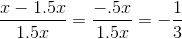Statement (1) is sufficient. (2) A customer with a reward card pays two thirds of any listed price. Let y be the listed price of a given article. A customer with reward card pays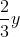. The percentage of discount is: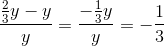Statement (2) is sufficient. Each Statement ALONE is sufficient. ### Example Question #1 : Dsq: Calculating Discounts What is the amount of tuition for a MBA degree at University X? (1) Students with a GMAT score above 700 receive a 50% discount on the tuition. (2) The average tuition paid by 5 students is$50,000, if only 2 of these students received a 50% discount.

Statement (2) ALONE is sufficient, but Statement (1) ALONE is not sufficient.

Statements (1) and (2) TOGETHER are not sufficient.

Statement (1) ALONE is sufficient, but Statement (2) ALONE is not sufficient.

Both statements TOGETHER are sufficient, but NEITHER statement ALONE is sufficient.

Each Statement ALONE is sufficient.

Statement (2) ALONE is sufficient, but Statement (1) ALONE is not sufficient.

Explanation:

(1) Students with a GMAT score above 700 receive a 50% discount on the tuition.

Statement (1) is not sufficient as we do not know how much half of the tuition is.

(2) The average tuition paid by 5 students is $50,000, if only 2 of these students received a 50% discount. Let x be the full amount of tuition for the MBA program.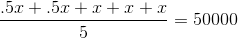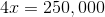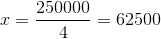Statement(2) Alone is sufficient. ### Example Question #91 : Word Problems How many dollars does Jane save when using a coupon to buy a purse at a local store? (1) The original price of the purse is$400.

(2) When using the coupon, Jane saves 25%.

Statement (2) ALONE is sufficient, but Statement (1) ALONE is not sufficient.

Each Statement ALONE is sufficient.

Statements (1) and (2) TOGETHER are not sufficient.

Statement (1) ALONE is sufficient, but Statement (2) ALONE is not sufficient.

Both statements TOGETHER are sufficient, but NEITHER statement ALONE is sufficient.

Both statements TOGETHER are sufficient, but NEITHER statement ALONE is sufficient.

Explanation:

Note that the question does not ask for the percentage of discount but the amount of money saved.

(1) The original price of the purse is $400. Statement (1) alone is not sufficient because it only gives the selling price prior to using the coupon. (2) When using the coupon, Jane saves 25%. Statement (2) alone is not sufficient. Even though we know the percentage of discount, we do not have the original price. However, combining both statements, we get the amount of money saved as: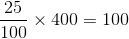Jane saved$100 by using the coupon.

Both statements TOGETHER are sufficient, but NEITHER statement ALONE is sufficient.

### Example Question #91 : Word Problems

A lawn-mower is initially offerred at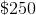. Its price is discounted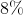off the full price. What is the final price?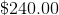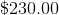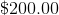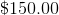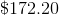Explanation:

If the initial price is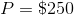, and the discount is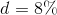, the final sale price is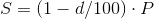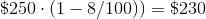### Example Question #1 : Dsq: Calculating Discounts

A lawn-mower is discountedoff the full price. A second discount ofis applied at the register. What is the final discount?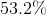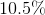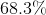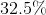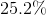Explanation:

If the initial price is P, and it is discounted twice at rates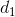and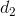, the final price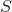is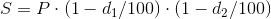Substituting values this is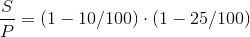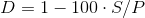or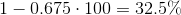### Example Question #1 : Discount

A lawn-mower is initially listed at. Its price is discounted off the full price some unknown amount,. An employee uses their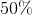discount at the register and is charged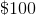.

What is the unknown initial discount,?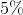Explanation:

The relationship between the list price,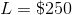, sale price,, and the employee's discount,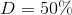, is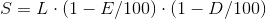Inserting values and re-organizing: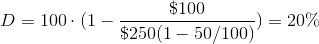### Example Question #92 : Data Sufficiency Questions

A lawn-mower is initially listed at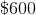. Its price is discountedoff the full price. How much does the customer save relative to the initial list price?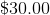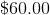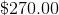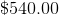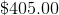Explanation:

If the initial price is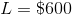, and the discount is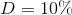, the savings are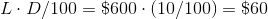### Example Question #2201 : Gmat Quantitative Reasoning

A lawn-mower is initially listed at.  Its price is discounted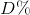off the full price.

In terms of, and, withexpressed in percent, what is the formula for the amount charged,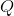at the register?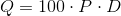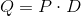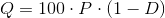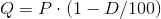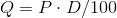Explanation:

The relationship between the list price,, the discountin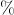, the price charged at the register is.

The factor ofrepresents the conversion fromto a decimal value.

← Previous 1

### All GMAT Math Resources